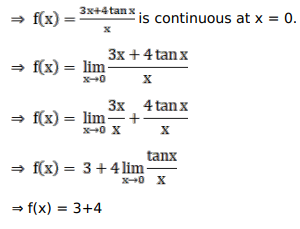# Solve this following

Question:

Mark $(\sqrt{)}$ against the correct answer in the following:

The value of $k$ for which $f(x)=\left\{\begin{array}{c}\frac{3 x+4 \tan x}{2}, \text { when } x \neq 0 \\ k, \text { when } x=0\end{array}\right.$ is continuous at $x=0$, is

A. 7

B. 4

C. 3

D. none of these

Solution:$\therefore K=7 .$